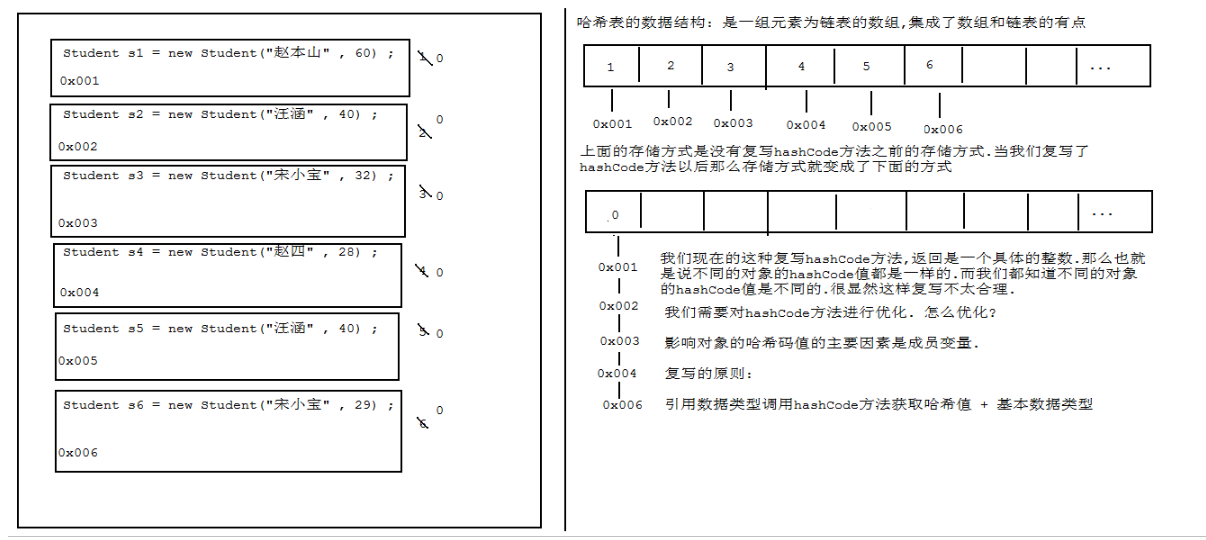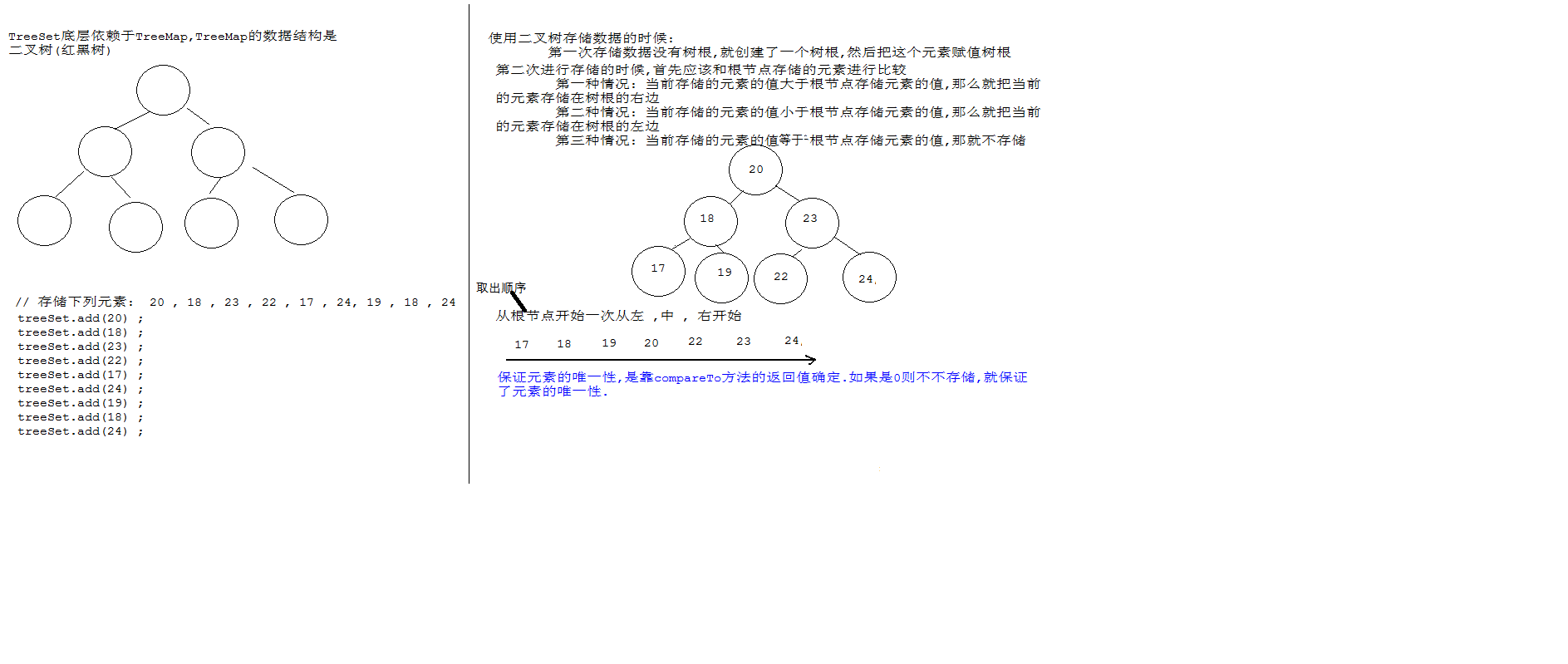# 泛型机制

### 泛型集合

  ArrayList<Student> list = new ArrayList<>();
//定义一个泛型为Student的迭代器，获取的元素类型为Student类型，
//无需向下转型
Iterator<Student> iterator = list.iterator();
while (iterator.hasNext()){
//定义一个泛型为Student的迭代器，获取的元素类型为Student类型，无需向下转型
Student next = iterator.next();
}


### 泛型类

  class Myclass<T,R>{
//定义一个不确定类型通过方法确定类型的私有变量
private T  t;
private R  r;
// 定义一个不确定类型调用方法才确定返回值类型的方法
public R getR(){return r;}
public void setR(R r){this.r=r;}
public T getT(){return t;}
public void setT(T t){this.t=t;}

}

Myclass<String,Integer> stringIntegerMyclass = new Myclass<>();
stringIntegerMyclass.setT("aaa");
String t = stringIntegerMyclass.getT();
stringIntegerMyclass.setR(100);
Integer r = stringIntegerMyclass.getR();


### 泛型方法

  test3 t3 = new test3();
//可以定义各种类型数据
t3.show(100);
t3.show("aaa");

//定义泛型方法
public<T>  void show(T num) {
System.out.println(num);
}
}


### 泛型接口

//定义泛型接口，子类实现接口时，可以明确接口上的泛型具体是什么类型
interface Myinterface<A,B>{
//定义一个传入参数类型与接口泛型相同的抽象方法
public abstract void test(A a);
public abstract B test1(B b);
}

//子类实现接口时，可以明确接口上的泛型具体是什么类型
class MyA implements Myinterface<Integer,String>{

@Override
public void test(Integer integer) {
System.out.println("Integer");
}
@Override
public String test1(String s) {
return s;
}
}
//采用匿名 内部类的这种方式，在创建接口的子类对象时，可以明确接口上的泛型具体是什么类型。
new Myinterface<Student,String>(){

@Override
public void test(Student student) {

}
@Override
public String test1(String s) {
return s;
}
};


### 泛型高级之通配符

? extends E: 向下限定，E及其子类
? super E: 向上限定，E及其父类

//泛型通配符，定义一个父类2个子类
class Animal{}
class Dog extends Animal{}
class  Cat extends Animal{}

ArrayList<?> objects = new ArrayList<Dog>();
ArrayList<?> objects1 = new ArrayList<Cat>();
ArrayList<?> objects2 = new ArrayList<Animal>();
//向上限定 类型为Cat的父类：Cat、Animal或者Object
ArrayList<? super Cat> list1 = new ArrayList<Animal>();
//向上限定，Animal的父类：Animal或者Object
ArrayList<? super Animal> list3 = new ArrayList<Animal>();
ArrayList<? super Animal> list4 = new ArrayList<Object>();
//向下限定 类型为Animal或子类
ArrayList<? extends Animal> list5 = new ArrayList<Cat>();
ArrayList<? extends Animal> list6 = new ArrayList<Animal>();


# 增强for

for(元素数据类型 变量 : 数组或者Collection集合) {
使用变量即可，该变量就是元素
}

int[] arr={2,3,4,5};
//for(容器中的元素的数据类型 变量名:容器名)
for (int i : arr) {
System.out.println(i);
}



# 可变参数

• a: 这里的变量其实是一个数组
• b: 如果一个方法有可变参数，并且有多个参数，那么，可变参数肯定是最后一个
int sum=add(1,2,3);

//可变参数的方法
private static int add(int a,int...b) {
int sum=a;
for (int i : b) {
sum+=i;
}
return sum;
}


# Arrays工具类的asList()

asList（）：

• 基本数据类型数组转换为集合存入的是地址值；传入引用数据类型数组转换集合存入的是每一个元素；传入多个引用类型数组转换集合存入多个引用类型数组的地址值
• 此方法得到的集合是Arrays的内部类，没有增删方法不支持这些操作，不能改变长度，只可以修改元素。
 //把一个数组转换集合。
int[] arr1={20,30,40};
//传的是一个基本类型的数组，他是把这个数组对象，放到集合中
List<int[]> ints = Arrays.asList(arr1);
//ints是arr1的地址值
System.out.println(ints.get(0));//20
Integer[] arr2 = {20, 30, 40};
//我传入的是一个包装类型的数组，他是吧数组中的元素，取出来放到集合
List<Integer> integers = Arrays.asList(arr2);
//integers就是一个集合  打印出这个集合
System.out.println(integers);
Integer[] arr3 = {20, 30, 40};
Integer[] arr4 = {20, 30, 400};
//如果你传入多个包装类型的数组，那么他是把多个数组对象，放到集合中。
List<Integer[]> integers1 = Arrays.asList(arr3, arr4);
//获取arr3的第一个元素
Integer integer = integers1.get(0);//20
//也可以这样得到一个集合。
// 注意：通过Arrays.asList() 这种方式的得到集合，不能再次改变集合的长度，也就是说，你不能增删元素。
List<Integer> integers2 = Arrays.asList(20, 30, 40);
System.out.println(integers2);



• 集合嵌套之ArrayList嵌套ArrayList
Student s1 = new Student("张三", 23);
Student s2 = new Student("李四", 23);
Student s3 = new Student("王五", 23);

Student s4 = new Student("赵六", 23);
Student s5 = new Student("田七", 23);
Student s6 = new Student("刘八", 23);

ArrayList<Student> Java_class = new ArrayList<>();

ArrayList<Student> Python_class = new ArrayList<>();

ArrayList<ArrayList<Student>> school = new ArrayList<>();



# set集合

### HashSet

• 底层数据结构是哈希表（JDK1.7 数组+链表 JDK1.8 优化 数组+链表+红黑树）
• 元素无序(存取元素的顺序不一致)且唯一（元素不允许重复）
• HashSet集合保证元素的唯一性，是靠元素重写 hashCode和equals() 方法来保证的，如果元素不重写，则无法保证元素的 唯一性。
原因如下：
• 首先根据对象的hashcode算出索引值，确定元素放在哈希表的位置；由于new对象的地址值不同，hashcode值不同在哈希表的位置也不同，如果将hashcode值固定为一个数，那么所有对象计算出的在哈希表中的位置是相同的，但是对象的地址值不同，就会在哈希表的同一个位置形成链表结构，那么会大量调用equals方法判断两个元素地址值是否相等，由于new出来的对象地址值都不相同，因此即使是相同的成员变量的对象，均是重复存储；

• 解决方案：通过成员变量值来重写hashcode方法，那么不同对象的成员变量值不同重写的hashcode计算出的在哈希表的位置就不同，就不会形成链式结构；如果不同对象计算出索引值相等，就再次调用重写的equals方法判断成员变量是否相等，不相等就存储；基本上通过第一层重写的hashcode方法就可以区分开不同的对象，无需调用equals方法，只有少机率会出现成员变量值不同单计算的索引值相等的情况，此时才需要调用重写的equals方法继续判断两个对象的成员变量值是否相等。

• 目的： 尽量减少链式结构与equals调用的次数；JDK1.8将链表数超过80会转为红黑树来判断，减少判断次数HashSet<Student> set1 = new HashSet<>();
for (Student student : set1) {
System.out.println(student);

}
/* Student{name='李四', age=24}
Student{name='scq', age=12}
Student{name='张三', age=12}
Student{name='王五', age=25}*/



student类如下：

import java.util.Objects;
public class Student
{
private String name;
private int age;

public String getName() {
return name;
}

public void setName(String name) {
this.name = name;
}

public int getAge() {
return age;
}

public void setAge(int age) {
this.age = age;
}

public Student() {}

public Student(String name, int age) {
this.name = name;
this.age = age;
}

@Override
public String toString() {
return "Student{" +
"name='" + name + '\'' +
", age=" + age +
'}';
}

@Override
public boolean equals(Object o)
{
if (this == o)
return true;
if (o == null || getClass() != o.getClass())
return false;
Student student = (Student) o;
return age == student.age && Objects.equals(name, student.name);
}

@Override
public int hashCode()
{
return Objects.hash(name, age);
}
}



Hashset对于集合的去重

ArrayList<Integer> list = new ArrayList<>();

HashSet<Integer> integers = new HashSet<>(list);
System.out.println(integers);//


 LinkedHashSet<Integer> integers1 = new LinkedHashSet<>();

for (Integer integer : integers1) {
System.out.println(integer);

}
/*  100
200
300*/



### TreeSet

TreeSet 底层数据结构是二叉树，元素有序唯一。Treeset对于储Integer类型的元素并遍历

 TreeSet<Integer> treeSet = new TreeSet<>();

for (Integer integer : treeSet) {
System.out.println(integer);
}
/*17
18
20
22
23
24 */


1、自然排序。如果new一个TreeSet对象的时候采用空参构造，就默认为是自然排序。你给集合当中添加Integer类型的数据时，就不会报错，这是因为Integer类当中实现了Comparable接口，重写了这个接口中的compare()。因此自定义的Student类如果想要添加到树集当中，就必须实现接口Comparable，并重写compare()。

2、比较器排序。采用有参构造，你给这个TreeSet对象的构造方法中传递一个比较器，实现接口Comparator，并重写compare()。

compare()的返回值代表元素应该添加到哪，后面添加的元素与根节点相比较，为0说明集合当中存在同样的对象，就存不进去了；因此如果你在代码当中将compare()返回值写死为0，就会出现一种现象，集合中只存进去根节点元素，其余都存不进去。为正数添加到红黑树的左边，为负数添加到红黑树的右边；如果compare()的返回值写成任意正负数，全部的元素都存储在了一个数组当中。

• 自然排序
学生类继承 Comparable接口并重写 compareTo 方法：
public class Student implements Comparable<Student> {
@Override
public int compareTo(Student s) {
/*按照姓名的长度来排序*/
//先比较姓名长度是否相等，返回正负数或0 排序
int num = this.name.length()-s.name.length();
//如果姓名长度相等判断姓名内容是否相等 ，姓名长度不相等说明是不同的对象直接返回num值给num2
int num2=num==0?this.name.compareTo(s.name):num;
//如果姓名长度和内容都相等判断年龄是否相等，否则返回num2
int num3=num2==0?this.age-s.age:num2;
return num3;
//根据年龄大小来排序
/*int num=this.age-s.age;
//如果年龄相同，并不能说明他们是同一个对象，还得比较姓名是否相同。
//compareTo返回一个数值 来作为二叉树的位置
int num2=num==0?this.name.compareTo(s.name):num;
return num2;*/
}

 //按照学生的年龄大小来排序
TreeSet<Student> treeSet2 = new TreeSet<>();
for (Student student : treeSet2) {
System.out.println(student.getName()+"=="+student.getAge());
}
/*张余生==20

*/

• 比较器排序
采用有参构造，给这个TreeSet对象的构造方法中传递一个比较器，实现接口Comparator，并重写compare()。
class MyCompareator implements Comparator<Student> {
@Override
public int compare(Student s1, Student s2) {
//安装年龄大小 来排序
int num = s1.getAge() - s2.getAge();
int num2 = num == 0 ? s1.getName().compareTo(s2.getName()) : num;
return num2;
}
}

TreeSet<Student> treeSet1 = new TreeSet<>(new MyCompareator());

for (Student student : treeSet1) {
System.out.println(student.getName() + "==" + student.getAge());
}

//方法二： 使用匿名内部类来传参
/* TreeSet(Comparator < ? super E > comparator)
构造一个新的空 TreeSet，它根据指定比较器进行排序。
Comparator 比较器*/

TreeSet<Student> treeSet3=  new TreeSet<>(new Comparator<Student>() {
@Override
public int compare(Student s1, Student s2) {
//安装年龄大小 来排序
int num = s1.getAge() - s2.getAge();
int num2 = num == 0 ? s1.getName().compareTo(s2.getName()) : num;
return num2;
}
});


  //使用比较器对于Integer类型的ArrayList集合进行排序
ArrayList<Integer> integers2 = new ArrayList<>();

integers2.sort(new Comparator<Integer>() {
@Override
public int compare(Integer o1, Integer o2) {
return o1-o2;
}
});
System.out.println(integers2);//[2, 23, 24, 25, 26]


 Integer[] arr={10,2,3,4};
Arrays.sort(arr, new Comparator<Integer>() {
@Override
public int compare(Integer o1, Integer o2) {
return o1-o2;
}
});
System.out.println(Arrays.toString(arr));//[2, 3, 4, 10]

10-181191
11-21301208-053439
05-01356
07-1933
08-081288
04-291993
05-25199
07-04410
09-011816